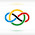### 2010 IMO SL #A5

Denote by $\mathbb{Q}^+$ the set of all positive rational numbers. Determine all functions $f : \mathbb{Q}^+ \mapsto \mathbb{Q}^+$ which satisfy the following equation for all $x, y \in \mathbb{Q}^+:$$f\left( f(x)^2y \right) = x^3 f(xy).$

The answer is $\boxed{f(x)=\frac{1}{x}}$.

We can easily plug in and check that this works.

We claim that $f$ is injective and $f(1)=1$. Note that $P(x,1)$ gives $f(f(x)^2)=x^2f(x)$. Then, whenever we will have $f(x)=f(y)$, then $$x^3 = \frac{f(f(x)^2)}{f(x)} = \frac{f(f(y)^2)}{f(y)} = y^3$$
$$\implies x=y.$$ So, $f$ is injective. Now $P(1,1)$ gives $$f(f(1)^2)=f(1) \implies f(1)^2=1 \implies f(1)=1.$$ So, we have proven our claim.

Next, we claim that $f(xy)=f(x)f(y)$. The original $P(x,y)$ is $f(f(x)^2y)=x^3f(xy)$. Also, $P(y,f(x)^2)$ gives $$f(f(y)^2f(x)^2)=y^3f(yf(x)^2) \implies f(yf(x)^2) = \tfrac{1}{y^3} f(f(y)^2f(x)^2).$$ Hence, we have $\tfrac{1}{y^3}f(f(y)^2f(x)^2) = x^3f(xy) \implies f(f(y)^2f(x)^2) = x^3y^3f(xy).$ Now $P(xy,1)$ gives $f(f(xy)^2)=x^3y^3f(xy)$, so $f(f(y)^2f(x)^2) = f(f(xy)^2) \implies f(x)^2f(y)^2 = f(xy)^2 \implies f(x)f(y)=f(xy),$ as desired by our claim.

Hence, combining our two claims, we have that $f$ is multiplicative.

Now using the multiplicativity, the original assertion can be written as $f(f(x))^2f(y)=x^3f(x)f(y)$, or $f(f(x))^2 = x^3 f(x)$. At this point, let $g(x)=xf(x)$. Note that $g$ is multiplicative as well, since $f$ is. It follows that $f(f(x)) = \frac{g(f(x))}{f(x)} = \frac{g(g(x)/x)}{g(x)/x} = \frac{g(g(x)) g(1/x)}{g(x)/x} = \frac{xg(g(x))}{g(x)^2}$. So $f(f(x)) = x^{3/2} \sqrt{f(x)} \implies \frac{xg(g(x))}{g(x)^2} = x^{3/2} \sqrt{g(x)/x} \implies g(g(x)) = g(x)^{5/2}.$So, $g^{k+1}(x) = g(x)^{5^k/2^k}$ for all $k \ge 1$. Unless we have $g(x)=1$, this will eventually become non-rational. Thus, $$xf(x)=g(x)=1 \implies f(x)=\frac{1}{x}$$ for all $x$, as desired. $\square$

1.Anonymous12/31/2021

f(x) = 0 is also solution to this equation

2.Hi @Anonymous

f(x)=0 is not a solution to this functional equation because f:Q+ ↦ Q+, which means that the outputs have to be positive rational numbers. However, 0 is not positive, so f(x)=0 is not a solution.

Thanks for the commenting on my blog, though!